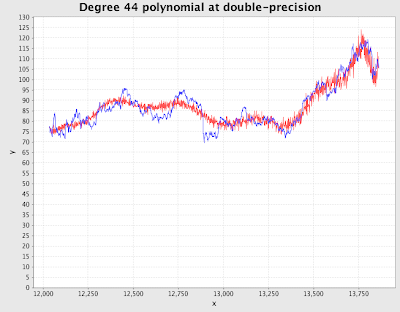## Monday, December 31, 2007

### Double (Im)precision

However, as I've long know but rarely contemplated, there if another form of floating point error that can cause significant problems. Standard floating point numbers only store so many digits of precision. The result is that if you add a very large number to a very small number, the very small number simply vanishes. Let me demonstrate:

```scala> 10000000000000000.0 + 1.0
res62: Double = 1.0E16
scala>
```

The 1.0 simply vanished, because standard double-precision floating point numbers don't have enough digits to store the 1.0 part of such a large number. Doubles are an approximation, and that's fine, because often times all we're approximating things, anyway, and the roughly 15 decimal digits of precisions provided by a double is plenty, right?

Well, actually, no. It depends on what you're doing with them. A month or so ago my father convinced me that instead of spending my free time playing with AI problems and studying fringe programming languages, I should do something useful like predict stock price movements. Of course I can use AI and fringe programming languages to do this...

The first thing I decided to do was to smooth out stock price history and make it continuous by fitting a polynomial. So I wrote a quick polynomial class, grabbed Jama (a Java matrix library), downloaded some data, wrote a function to search for the appropriate degree, and this is what I got (supposedly with about 4% error):Hmmm...that looks kind of funny. The blue line is the actual price. The red line is the polynomial, which has a degree of 44. That's a rather large degree, but certainly not enough to generate all those squiggles. Those squiggles are an artifact of double precision numbers not being precise enough to be used in calculating a degree-44 polynomial. They don't work that well for the matrix calculations that produce the polynomial, either.

I think that red squiggly line is a very good illustration of what happens when you don't pay enough attention to how your software works under-the-hood. Anyway, here's what the results look like using floating point numbers (courtesy of JScience) with 80 digits of precision (keeping the error at about 4%).Looks a lot better, doesn't it? One interesting thing is that the resulting polynomial has a much, much lower degree than what was produced by the same algorithm using double precision numbers. With double precision numbers, 4% error was about as good as it could get with this dataset. However, using the higher-precision numbers it can go much lower. Below is a graph at about 2% error:At this point you might be wondering why anyone would use double precision numbers for this type of thing, or perhaps thinking I'm a moron for trying. I certainly felt a little stupid. But using doubles is relatively common. Matlab and everyone's favorite Excel use doubles. Many of the other toolkits and libraries that I found do as well.

Overall I think I'm either missing something (because the use of doubles is so common), or I find this very frightening. I'm not an expert in this area. I know the doubles use much, much less memory and computational resources. They are also "just there" in pretty much all programming environments, so they are the default. Making arbitrary precision floating points work right wasn't a cakewalk, either. I spent the better part of a day tracing through my code, only to discover a bug in JScience. Also, once you have everything working, you have to figure out what precision to use. The default of 20 digits precision in JScience wasn't noticeably better than regular doubles.

Sphere: Related Content

Anonymous said...

You may want to try evaluating the polynomial using a different form. For example, given the polynomial:

A*x^3 + B*x^2 + C*x + D

one is often tempted to just enter it "as is". However it can also be expressed this way:

((A*x+B)*x+C)*x+D

Generally speaking polynomials often work better using floating point this alternate way. Some programming languages/systems know this and convert the expression before evaluating it.

Erik Engbrecht said...

Yes, I thought about making that change, and probably will because my current implementation is really naive. For anyone curious there's some information about it here.

The thing is worrying about how expressions involving floating points are evaluated makes floating point numbers be an extremely leaky abstraction, and one that most people don't spend a lot of time thinking about.